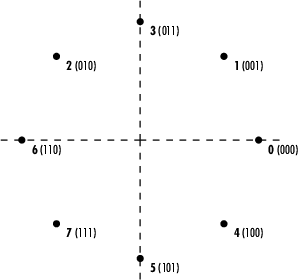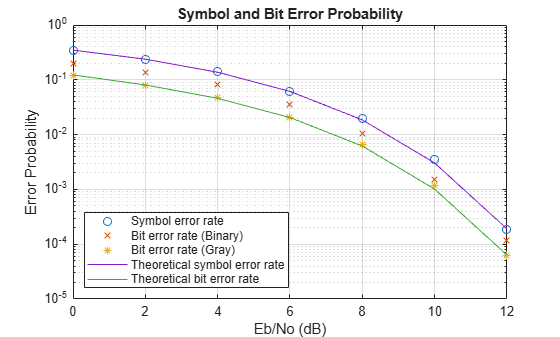Documentation

## Gray Coded 8-PSK

This example shows a communications system with Gray-coded 8-ary phase shift keying (8-PSK) modulation using communications related System objects. Gray coding is a technique that multilevel modulation schemes often use to minimize the bit error rate. It consists of ordering modulation symbols so that the binary representations of adjacent symbols differ by only one bit.

### Introduction

This example modulates data using the 8-PSK method. The data passes through an AWGN channel, and is demodulated using an 8-PSK demodulator. An error rate calculator System object measures the symbol and bit error rates.

In this communications system, the PSK Modulator System object:

• Accepts binary-valued inputs that represent integers between 0 and M – 1. M is the modulation order and is equal to 8 for 8-PSK modulation.

• Maps binary representations to constellation points using Gray-coded ordering.

• Produces unit-magnitude complex phasor outputs, with evenly spaced phases between 0 and 2π(M – 1)/M.

The following table indicates the relationship between binary representations in the input and phasors in the output. The second column of the table is an intermediate representation that the System object uses in its computations.

Modulator InputGray-Coded OrderingModulator Output
`000`0`exp`(0)
`001`1`exp`(jπ/4)
`010`3`exp`(j3π/4)
`011`2`exp`(jπ/2) = `exp`(j2π/4)
`100`7`exp`(j7π/4)
`101`6`exp`(j3π/2) = `exp`(j6π/4)
`110`4`exp`() = `exp`(j4π/4)
`111`5`exp`(j5π/4)

The table below sorts the first two columns from the previous table, according to the output values. This sorting makes it clearer that there is only a 1 bit difference between neighboring symbols. In the following figure, notice that the numbers in the second column of the table appear in counterclockwise order.

Modulator OutputModulator Input
`exp`(0)`000`
`exp`(/4)`001`
`exp`(/2) = `exp`(j2π/4)`011`
`exp`(j3π/4)`010`
`exp`() = `exp`(j4π/4)`110`
`exp`(j5π/4)`111`
`exp`(j3π/2) = `exp`(j6π/4)`101`
`exp`(j7π/4)`100`### Initialization

This section of the code initializes the system variables. It also creates and configures the System objects used in this example.

Set the modulation order to 8 for 8-PSK modulation. Run the simulation until either the specified maximum number of bit errors (maxNumErrs) or the maximum number of bits (maxNumBits) is reached. This simulation iterates over a number of bit energy to noise power spectral density Eb/No values.

```M = 8; % Modulation order SamplesPerFrame = 10000; % Symbols processed for each iteration of the % stream processing loop % Initialize variables used to determine when to stop processing bits maxNumErrs=100; maxNumBits=1e8; % Since the AWGN Channel as well as the RANDI function uses the default % random stream, the following commands are executed so that the results % will be repeatable, i.e. same results will be obtained for every run of % the example. The default stream will be restored at the end of the % example. prevState = rng; rng(529558);```

Create an integer to bit converter (hInt2Bit) and a bit to integer converter (hBit2Int) System object to convert the randomly generated integer data to bits and the demodulated data bits back to integers

```hInt2Bit = comm.IntegerToBit('BitsPerInteger',log2(M), ... 'OutputDataType','uint8'); hBit2Int = comm.BitToInteger('BitsPerInteger',log2(M), ... 'OutputDataType','uint8');```

Create and configure a PSK modulator (hMod) System object to map the binary input data to an 8-PSK gray coded constellation as well as a matching PSK demodulator (hDemod) System object

```hMod = comm.PSKModulator('ModulationOrder',M, ... 'SymbolMapping','gray', ... 'PhaseOffset',0, ... 'BitInput',true); hDemod = comm.PSKDemodulator('ModulationOrder',M, ... 'SymbolMapping','gray', ... 'PhaseOffset',0, ... 'BitOutput',true, ... 'OutputDataType','uint8', ... 'DecisionMethod','Hard decision');```

Create an AWGN channel System object to add additive white Gaussian noise to the modulated signal. The noise method is appropriately selected so it specifies the bit energy to noise power spectral density in the stream processing loop. Because the PSK modulator generates symbols with 1 Watt of power, the signal power property of the AWGN channel is also set to 1.

```hChan = comm.AWGNChannel('NoiseMethod','Signal to noise ratio (Eb/No)', ... 'BitsPerSymbol',log2(M), ... 'SignalPower',1);```

Create a symbol error rate calculator (hSymError) and a bit error rate calculator (hBitError) System object to compare the demodulated integer and bit data with the original source data. This comparison yields symbol error and bit error statistics. The output of the error rate calculator System object is a three-element vector containing the calculated error rate, the number of errors observed, and the amount of data processed. The simulation uses the three-element vector generated by hBitError to determine when to stop the simulation.

```hSymError = comm.ErrorRate; hBitError = comm.ErrorRate;```

### Stream Processing Loop

This section of the code calls the processing loop where data is gray coded, modulated, and demodulated using 8-PSK modulation. The loop simulates the communications system for Eb/No values in the range 0dB to 12dB in steps of 2dB.

```% For each Eb/No value, simulation stops when either the maximum number of % errors (maxNumErrs) or the maximum number of bits (maxNumBits) processed % by the bit error rate calculator System object is reached. EbNoVec = 0:2:12; % Eb/No values to simulate SERVec = zeros(size(EbNoVec)); % Initialize SER history BERVec = zeros(size(EbNoVec)); % Initialize BER history for p = 1:length(EbNoVec) % Reset System objects reset(hSymError); reset(hBitError); hChan.EbNo = EbNoVec(p); % Reset SER / BER for the current Eb/No value SER = zeros(3,1); % Symbol Error Rate BER = zeros(3,1); % Bit Error Rate while (BER(2)<maxNumErrs) && (BER(3)<maxNumBits) % Generate random data txSym = randi([0 M-1], SamplesPerFrame, 1, 'uint8'); txBits = step(hInt2Bit, txSym); % Convert symbols to bits tx = step(hMod, txBits); % Modulate rx = step(hChan, tx); % Add white Gaussian noise rxBits = step(hDemod, rx); % Demodulate rxSym = step(hBit2Int, rxBits); % Convert bits back to symbols % Calculate error rate SER = step(hSymError, txSym, rxSym); % Symbol Error Rate BER = step(hBitError, txBits, rxBits); % Bit Error Rate end % Save history of SER and BER values SERVec(p) = SER(1); BERVec(p) = BER(1); end```

### Cleanup

Restore the default stream.

`rng(prevState)`

### Conclusions

Analyze the data that the example produces and compare theoretical performance with simulation performance. The theoretical symbol error probability of MPSK is

`${P}_{E}\left(M\right)=erfc\left(\sqrt{\frac{{E}_{s}}{{N}_{0}}}\mathrm{sin}\left(\frac{\pi }{M}\right)\right)$`

where erfc is the complementary error function, Es/N0 is the ratio of energy in a symbol to noise power spectral density, and M is the number of symbols.

To determine the bit error probability, convert the symbol error probability, PE, to its bit error equivalent. There is no general formula for the symbol to bit error conversion. Nevertheless, upper and lower limits are easy to establish. The actual bit error probability, Pb, can be shown to be bounded by

`$\frac{{P}_{E}\left(M\right)}{{\mathrm{log}}_{2}M}\le {P}_{b}\le \frac{M/2}{M-1}{P}_{E}\left(M\right)$`

The lower limit corresponds to the case where the symbols have undergone Gray coding. The upper limit corresponds to the case of pure binary coding.

The following script plots the simulated symbol error rates (SERVec) and bit error rates (BERVec) together with the theoretical symbol error and bit error probabilities.

Calculate theoretical error probabilities.

`[theorBER, theorSER] = berawgn(EbNoVec, 'psk', M, 'nondiff');`

Plot the results.

```figure; semilogy(EbNoVec,SERVec,'o', EbNoVec,BERVec,'*', ... EbNoVec,theorSER,'-', EbNoVec,theorBER,'-'); legend ( 'Symbol error rate', 'Bit error rate', ... 'Theoretical Symbol error rate', 'Theoretical Bit error rate', ... 'Location','SouthWest'); xlabel ( 'Eb/No (dB)' ); ylabel( 'Error Probability' ); title ( 'Symbol and Bit Error Probability' ); grid on;```As a further exercise, you can compare Gray coding with pure binary coding by modifying the PSK modulator and PSK demodulator System objects so that their constellation ordering parameters are 'Binary' instead of 'Gray'. Setting this property and re-running the simulation should generate results similar to the following: